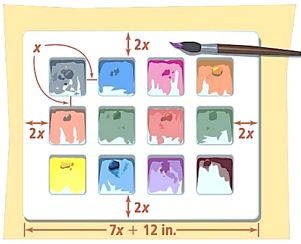Mathematics
Easy

Question

# A paint tray has an area of 42x2 + 135x + 108 square in. The square paint compartments that are all the same size and spaced evenly, though the space along the edge of the tray is twice as wide as the space between squares.What is the width of the paint tray?

## 6x – 85x + 76x + 95x + 11Hint:

## The correct answer is: 6x + 9

### To find the width, lets factor the area trinomial.42x2 + 135x + 108Take out 3.3(14x2 + 45x + 36). So, first factor 14x2 + 45x + 36.a = 14, b = 45, c = 36ac = 14×36 = 504Factors of 504 are 21 and 24.21 + 24 = 4514x2 + 21x + 24x + 36 = 7x(2x + 3) + 12(2x + 3) = (7x + 12)(2x + 3)42x2 + 135x + 108= 3(7x + 12)(2x + 3) = (7x + 12)(6x + 9)So, length is 7x + 12 in. and width is 6x + 9 in.Hence, the correct option is C.

Area of a rectangle= length × breadth sq. units.

### Related Questions to study#### With Turito Foundation.#### Get an Expert Advice From Turito.